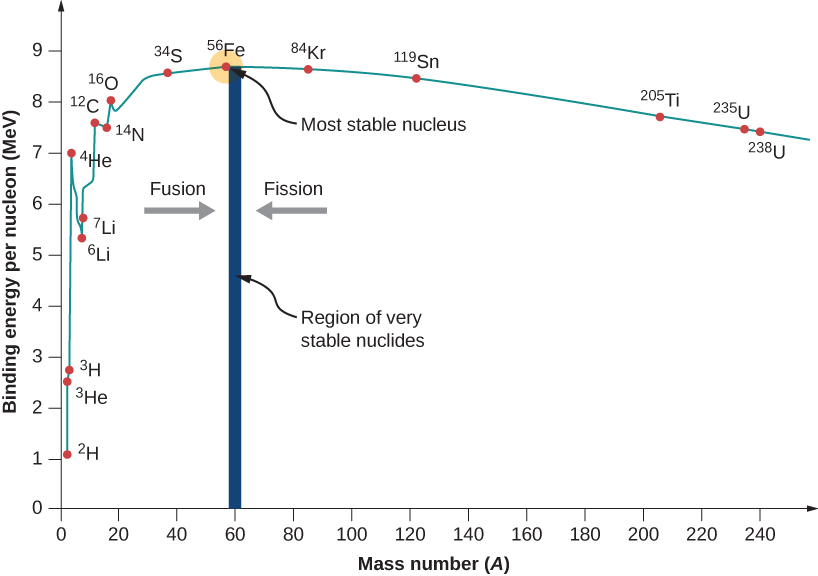Nucleons are attracted to each other by the strong nuclear force. The nuclear binding energy is the amount of energy needed to disassemble a nucleus into its constituent parts.

For nuclear reactions, the total binding energy of the resulting elements must be greater than that of the starting element.

## Mass Defect §

The mass of an atomic nucleus is less than the sum of the individual masses of the free constituent protons and neutrons. The difference in mass can be calculated by the Einstein equation, and the energy is equivalent to the nuclear binding energy. In the equation, is the mass defect.

## Binding Energy §

Nuclear Fusion uses lighter elements, such as hydrogen and helium, which are in general more fusible; while the heavier elements, such as uranium, thorium and plutonium, are more fissionable.

The inverted curve is due to two opposing forces:

1. the nuclear force, a manifestation of the strong interaction, which holds protons and neutrons tightly together in the atomic nucleus; and
2. the Coulomb force, which causes positively charged protons in the nucleus to repel each other.

Under most conditions, the Coulomb force dominates. However, when accelerated to high enough speeds, nuclei can overcome this electrostatic repulsion and be brought close enough such that the attractive nuclear force is greater than the repulsive Coulomb force.